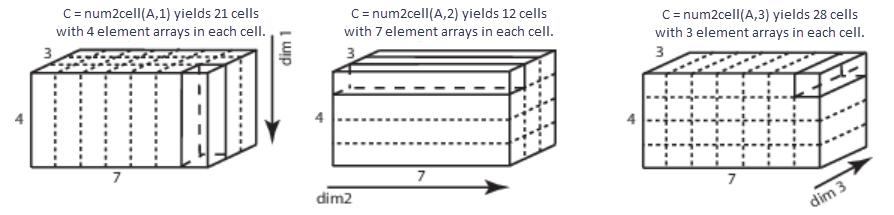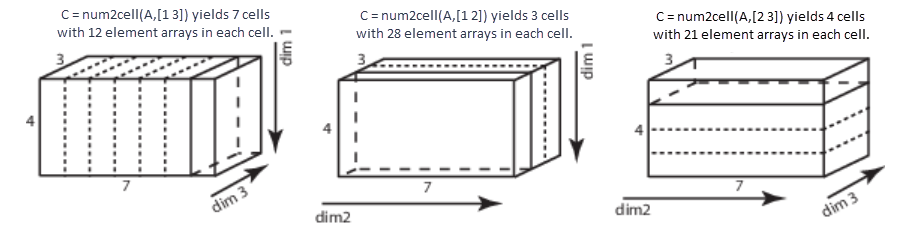# num2cell

Convert array to cell array with consistently sized cells

## Syntax

``C = num2cell(A)``
``C = num2cell(A,dim)``

## Description

example

````C = num2cell(A)` converts array `A` into cell array `C` by placing each element of `A` into a separate cell in `C`.The `num2cell` function converts an array that has any data type—even a nonnumeric type.```

example

````C = num2cell(A,dim)` splits the contents of `A` into separate cells of `C`, where `dim` specifies which dimensions of `A` to include in each cell. `dim` can be a scalar or a vector of dimensions. For example, if `A` has 2 rows and 3 columns, then: `num2cell(A,1)` creates a 1-by-3 cell array `C`, where each cell contains a 2-by-1 column of `A`.`num2cell(A,2)` creates a 2-by-1 cell array `C`, where each cell contains a 1-by-3 row of `A`.`num2cell(A,[1 2])` creates a 1-by-1 cell array `C`, where the cell contains the entire array `A`. ```

## Examples

collapse all

Place all elements of a numeric array into separate cells.

`a = magic(3)`
```a = 3×3 8 1 6 3 5 7 4 9 2 ```
`c = num2cell(a)`
```c=3×3 cell array {} {} {} {} {} {} {} {} {} ```

Place individual letters of a word into separate cells of an array.

`a = ['four';'five';'nine']`
```a = 3x4 char array 'four' 'five' 'nine' ```
`c = num2cell(a)`
```c = 3x4 cell {'f'} {'o'} {'u'} {'r'} {'f'} {'i'} {'v'} {'e'} {'n'} {'i'} {'n'} {'e'} ```

Generate a 4-by-3-by-2 numeric array, and then create a 1-by-3-by-2 cell array of 4-by-1 column vectors.

```A = reshape(1:12,4,3); A(:,:,2) = A*10```
```A = A(:,:,1) = 1 5 9 2 6 10 3 7 11 4 8 12 A(:,:,2) = 10 50 90 20 60 100 30 70 110 40 80 120 ```
`C = num2cell(A,1)`
```C = 1x3x2 cell array C(:,:,1) = {4x1 double} {4x1 double} {4x1 double} C(:,:,2) = {4x1 double} {4x1 double} {4x1 double} ```

Each 4-by-1 vector contains elements from along the first dimension of `A`:

`C{1}`
```ans = 4×1 1 2 3 4 ```

Create a 4-by-1-by-2 cell array of 1-by-3 numeric arrays.

`C = num2cell(A,2)`
```C = 4x1x2 cell array C(:,:,1) = {[ 1 5 9]} {[2 6 10]} {[3 7 11]} {[4 8 12]} C(:,:,2) = {[ 10 50 90]} {[20 60 100]} {[30 70 110]} {[40 80 120]} ```

Each 1-by-3 row vector contains elements from along the second dimension of `A`:

`C{1}`
```ans = 1×3 1 5 9 ```

Finally, create a 4-by-3 cell array of 1-by-1-by-2 numeric arrays.

`C = num2cell(A,3)`
```C=4×3 cell array {1x1x2 double} {1x1x2 double} {1x1x2 double} {1x1x2 double} {1x1x2 double} {1x1x2 double} {1x1x2 double} {1x1x2 double} {1x1x2 double} {1x1x2 double} {1x1x2 double} {1x1x2 double} ```

Each 1-by-1-by-2 vector contains elements from along the third dimension of `A`:

`C{1}`
```ans = ans(:,:,1) = 1 ans(:,:,2) = 10 ```

Create a cell array by combining elements into numeric arrays along several dimensions.

```A = reshape(1:12,4,3); A(:,:,2) = A*10```
```A = A(:,:,1) = 1 5 9 2 6 10 3 7 11 4 8 12 A(:,:,2) = 10 50 90 20 60 100 30 70 110 40 80 120 ```
`c = num2cell(A,[1 3])`
```c=1×3 cell array {4x1x2 double} {4x1x2 double} {4x1x2 double} ```

Each 4-by-1-by-2 array contains elements from along the first and third dimension of `A`:

`c{1}`
```ans = ans(:,:,1) = 1 2 3 4 ans(:,:,2) = 10 20 30 40 ```
`c = num2cell(A,[2 3])`
```c=4×1 cell array {1x3x2 double} {1x3x2 double} {1x3x2 double} {1x3x2 double} ```

## Input Arguments

collapse all

Input, specified as any type of multidimensional array.

Data Types: `double` | `single` | `int8` | `int16` | `int32` | `int64` | `uint8` | `uint16` | `uint32` | `uint64` | `logical` | `char` | `string` | `struct` | `cell` | `categorical` | `datetime` | `duration` | `calendarDuration` | `function_handle`

Dimension of `A`, specified as a positive integer or a vector of positive integers. `dim` must be between 1 and `ndims`(`A`).

Elements do not need to be in numeric order. However, `num2cell` permutes the dimensions of the arrays in each cell of `C` to match the order of the specified dimensions.

Data Types: `double` | `single` | `int8` | `int16` | `int32` | `int64` | `uint8` | `uint16` | `uint32` | `uint64`

## Output Arguments

collapse all

Resulting array, returned as a cell array. The size of `C` depends on the size of `A` and the values of `dim`.

• If `dim` is not specified, then `C` is the same size as `A`.

• If `dim` is a scalar, then `C` contains `numel(A)/size(A,dim)` cells. If `dim` is 1 or 2, then each cell contains a column or row vector, respectively. If `dim` > 2, then each cell contains an array whose `dim`th dimensional length is `size(A,dim)`, and whose other dimensions are all singletons.

For example, given a 4-by-7-by-3 array, `A`, this figure shows how `num2cell` creates cells corresponding to `dim` values of `1`, `2`, and `3`.• If `dim` is a vector containing `N` values, then `C` has `numel(A)/prod([size(A,dim(1)),...,size(A,dim(N))])` cells. Each cell contains an array whose `dim``(i)`th dimension has a length of `size(A,dim(i))` and whose other dimensions are singletons.

For example, given a 4-by-7-by-3 array, you can specify `dim` as a positive integer vector to create cell arrays of different dimensions.Data Types: `cell`

## Tips

• To convert the cell array `C` back to an array, use the `cell2mat` function, or use the syntax `cat(dim,C{:})` where `dim` specifies the dimensions.

## Version History

Introduced before R2006a